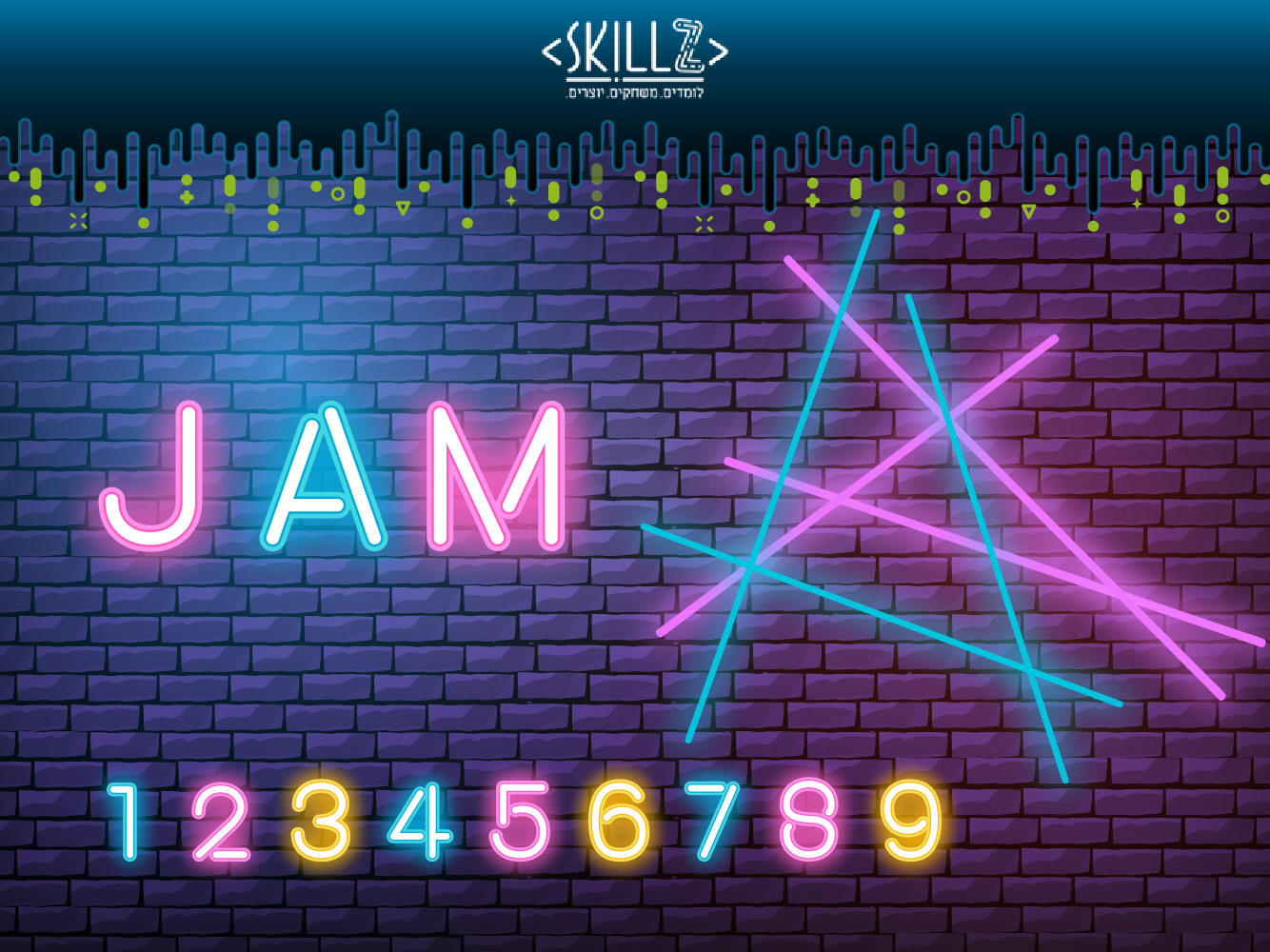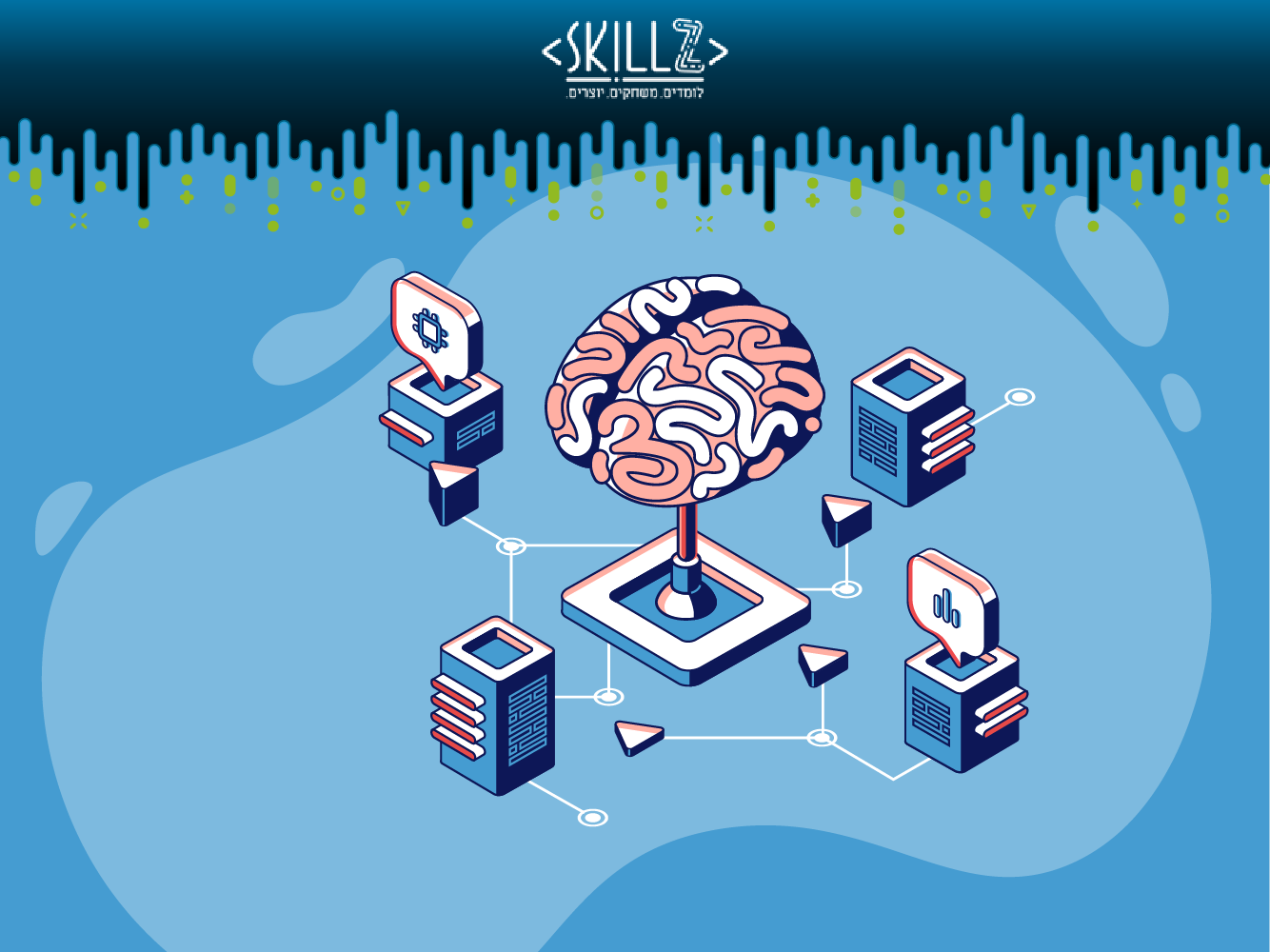# Shannon's Entropy

### This MOOC teaches the math behind Shannon's entropy

This miniMOOC teaches the math behind Shannon's entropy. It was created by Dr. Rivki Gadot (Open University , LevAcademic Center) & Dvir Lanzberg (the lecturer). It was made for undergraduate students, but we believe that anyone with a basic knowledge in calculus and algebra can get over the math in this miniMOOC.

Shannon started by asking what is "information" and how it can be measured. Instead of giving a definition, he claimed that any function that measures information must have three properties, and proved that there is a single function that meets these three properties. This function become to be known as, Shannon's entropy.

There are six short clips in this miniMOOC. Each clip is accompanied by exercises or a quiz that let you deepen your understanding. The topics of the six clips are:

1. The problem - states what was the problem that Shannon tried to solve.
2. The solution - introduces Shannon's entropy.
3. The three properties - explains what are the three properties that every function for the amount of information, must have.
4. Equal probabilities - proves that Shannon's entropy is the only function that has the three properties, if the events' probabilities were equal.
5. Rational probabilities - proves that Shannon's entropy is the only function that has the three properties, if the events' probabilities were rational numbers.
6. Real probabilities - proves that Shannon's entropy is the only function that has the three properties, if the events' probabilities were real numbers.

The clips' presentation in PPTX format, and in PDF format (including lecturer's note).

#### Information and surprise

This clip states what was the problem that Shannon tried to solve.

1. How much surprise is there in finding out the outcome of a coin toss?
2. How much surprise is there in finding out which permutation of an array is sorted?
3. How much surprised can we get by knowing the weather in Tel-Aviv in August?

After watching the clip, try to solve try to solve these problems: Part 1 - Questions.

&nbsp

#### Entropy

This clip introduces Shannon's entropy.

So given n events how much information (the surprise, the entropy) is there in knowing which event happened?

&nbsp

&nbsp

After watching the clip, try to solve these problems: Part 2 - Questions

&nbsp

#### Shannon required three properties of H:

This clip explains what are the three properties that every function for the amount of information, must have:

1. H should be continuous in the pi
2. H(1/n,…,1/n) should be a monotonic increasing function of n
3. If a choice be broken down into two successive choices, the original H should be the weighted sum of the individual values of H

After watching the clip, try to solve these problems: Part 3 - Questions

&nbsp

#### Lets start by considering n events with equal probabilities.

This clip proves that Shannon's entropy is the only function that has the three properties, if the events' probabilities were equal.

1. What are the other options?
2. What does it mean equal probabilities?
3. What is the value of H in this case?

After watching the clip, try to solve these problems: Part 4 - Questions

&nbsp

#### Now consider n events with rational probabilities.

This clip proves that Shannon's entropy is the only function that has the three properties, if the events' probabilities were rational numbers.

1. Is this the general case?
2. What is the value of H in this case?

&nbsp

After watching the clip, try to solve these problems: Part 5 - Questions

&nbsp

#### Eventually consider n events with real probabilities.

This clip proves that Shannon's entropy is the only function that has the three properties, if the events' probabilities were rational numbers.

1. Is this the general case?
2. What is the value of H in this case?

&nbsp

After watching the clip, try to solve these problems: Part 6 - Questions

&nbsp

#### Before we say goodbye...

###### Some Questions We Did Not Discuss (and You May Want to Research Yourself)
1. Who was Shannon? What else did he discovered and invented?
2. What is the meaning of "Entropy"?
3. Why is Shannon's entropy so important?

&nbsp

#### Materials

The course materials are accessible in a concentrated manner.
One can download the presentation that accompanies the course at PPTX format or at PDF format (including the lecturer's note), and for each part one can download the video, the questions and the answers:

### miniMOOCs נוספים

#### 15#### מגדלי הנוי - miniMOOC#### Shanon Entropyאודות הקורס
מטרת הקורס להדגים רדוקציה בין בעיות, כלומר איך ניתן להמיר בעיה אחת לבעיה אחרת. רדוקציה יכולה לסייע לפתור בעיה בעזרת פתרון בעיה אחרת.
הקורס פותח ע"י דביר לנצברג והוא מורכב משלושה סרטונים, שאלונים ושאלות לחשיבה.

מרצה: דביר לנצברג
שפה: עברית

למעבר ל mini MOOC

אודות הקורס
מטרת הקורס להדגים כיצד כתיבת אלגוריתם לפתרון בעיה
מאפשר להגיע לתובנות לגבי הבעיה ופתרונה.
הקורס פותח ע"י דביר לנצברג והוא מורכב משלושה סרטונים, שאלונים ושאלות לחשיבה.

מרצה: דביר לנצברג
שפה: עברית

למעבר ל mini MOOC

This miniMOOC teaches the math behind Shannon's entropy. The miniMOOC created by Rivki Gadot & Dvir Lanzberg and it requires a basic knowledge in calculus and algebra .

Lecturer - Dvir Lanzberg
Language - English

אולי ימצא חן בעיניך#### חשיבה חישובית - VOD

24 במרץ 2020

ספריית ה-VOD של Skillz. סרטוני הדרכה בעברית ובערבית

קרא עוד#### אלופי הבית - מדריך למשתמש

15 במרץ 2020

הכירו את תחרות אלופי הבית

קרא עוד#### العاب التاج - VOD

24 במרץ 2020

مكتبة الدروس المسجلة - VOD لألعاب التاج

קרא עוד